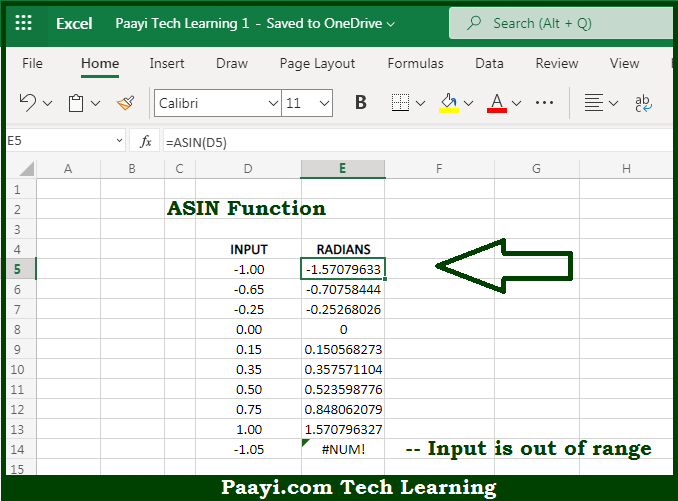# Learn How to Use Microsoft Excel ASIN Function

Written by | 0 Comments | 714 Views

In this article, you will learn how to use the Microsoft Excel ASIN function and its prime function in Microsoft Excel. You will also get to know the Microsoft Excel ASIN function return value and syntax with the help of some examples.

Microsoft Excel ASIN Function

The main function of the Microsoft Excel ASIN function is to get the inverse sine of a value in radians. That implies, with the help of the ASIN function you can able to return the inverse sine of the number. It should be noted that the ASIN function is the inverse of the SIN function and you need to input the values in the range of -1 to 1. So, with the help of the ASIN function, you can able to get inverse sine values of a value, in radians.

Return Value of ASIN Function

The return value will be the angle in radians.

Syntax of ASIN Function

=ASIN(number)

Where the arguments:

• number: This is the number or value of which you want to get the inverse sine, and it should be between the range of -1 and 1.

## How to Use Microsoft Excel ASIN Function?So we know that Microsoft Excel ASIN function you can able to get the inverse sine of a value in radians. That implies, with the help of the ASIN function you can able to return the inverse sine of the number. It should be noted that the ASIN function is the inverse of the SIN function and you need to input the values in the range of -1 to 1. So, with the help of the ASIN function, you can able to get inverse sine values of a value, in radians.